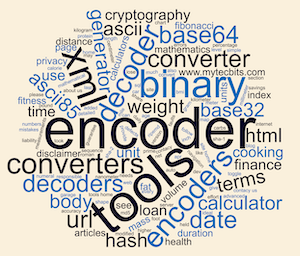# Base16 / Hexadecimal Encoder Tool

Share:

Enter or paste your text in the first text box and click the Encode button.

The text will be encoded for base 16 and appears in the second box below.

*Base16, also known as hexadecimal, is a numbering system that uses 16 symbols to represent numbers. These symbols are typically the numbers 0-9 and the letters A-F.

A Base16 encoder is a tool that converts data from its original binary format into Base16 format. The encoding process involves breaking down the binary data into 4-bit chunks and representing each chunk as a Base16 symbol.

For example, To encode the ASCII string "MyTecBits" in Base16, we first need to convert it to its binary representation. Each character in the string is represented by a unique ASCII code, which is itself represented in binary using 8 bits. We can use the following table to convert each character to its binary representation:

CharacterASCII Code (Decimal)ASCII Code (Binary)
M7701001101
y12101111001
T8401010100
e10101100101
c9901100011
B6601000010
i10501101001
t11601110100
s11501110011

Next, we need to combine the binary representations of each character into a single binary string. Doing so gives us the following binary representation for the string "MyTecBits":

0100110101111001010101000110010101100011010000100110100101110100011100110

To encode this binary string in Base16, we need to divide it into groups of 4 bits (since each Base16 symbol represents 4 bits). Doing so gives us the following groups:

0100 1101 0111 1001 0101 0100 0110 0101 0110 0011 0100 0010 0110 1001 0111 0100 0111 0011

We can then use a Base16 lookup table to convert each group of 4 bits to its corresponding Base16 symbol:

00000
00011
00102
00113
01004
01015
01106
01117
10008
10019
1010A
1011B
1100C
1101D
1110E
1111F

Using this table, we can convert each group of 4 bits to its corresponding Base16 symbol, giving us the following Base16-encoded string:

4D7954656342697473

This is the Base16-encoded representation of the original string "MyTecBits".

Base16 encoding is often used in computing systems to represent binary data as plain text for easier transmission and storage. It is commonly used in digital certificates, network protocols, and in encoding data for use in URLs.

In summary, a Base16 encoder is a tool that converts binary data into Base16 format by breaking it down into 4-bit chunks and representing each chunk as a Base16 symbol. Base16 encoding is widely used in computing systems to represent binary data as plain text for easier transmission and storage.